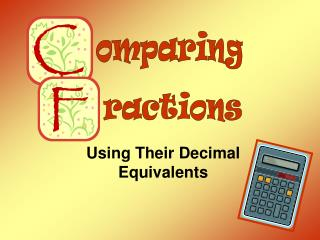DownloadDownload Presentationomparing

# omparing

Télécharger la présentation## omparing

- - - - - - - - - - - - - - - - - - - - - - - - - - - E N D - - - - - - - - - - - - - - - - - - - - - - - - - - -
##### Presentation Transcript

1. omparing ractions Using Their Decimal Equivalents

2. Comparing FractionsUsing Their Decimal Equivalents

3. Comparing FractionsUsing Their Decimal Equivalents

4. Example #1 ~ Compare these fractions. 4 ÷ 5 = 3 ÷ 7 = 0.8 0.428 Next, compare the decimals.

5. Comparing Decimals

6. Comparing Decimals

7. Comparing Decimals

8. Example #1 ~ Compare these fractions. 0.8 0.428 < < = The greater than symbol can be placed between these fractions.

9. Which sign belongs between the two fractions? 6 ÷ 7 = 4 ÷ 9 = < 0.857 0.444

10. Which sign belongs between the two fractions? 0.857 0.444 < < = greater than

11. Change Each Fraction to a Decimal 0.75 0.83 Five-sixths is the greater fraction.

12. Comparing FractionsUsing Their Decimal Equivalents Can you solve these problems?

13. 0.428 0.444 < A

14. 0.444 0.375 < B

15. 0.4 0.571 < C

16. 0.5 0.5 = D

17. 0.6 0.666 < E

18. 0.875 0.714 < F

19. 0.4 0.5 < G

20. 0.857 0.666 < H

21. 0.666 0.666 = I

22. 0.285 0.428 < J

23. 0.5 0.333 < K

24. 0.375 0.333 < L

25. D

26. 0.166 0.375 < M

27. 0.444 0.5 < N